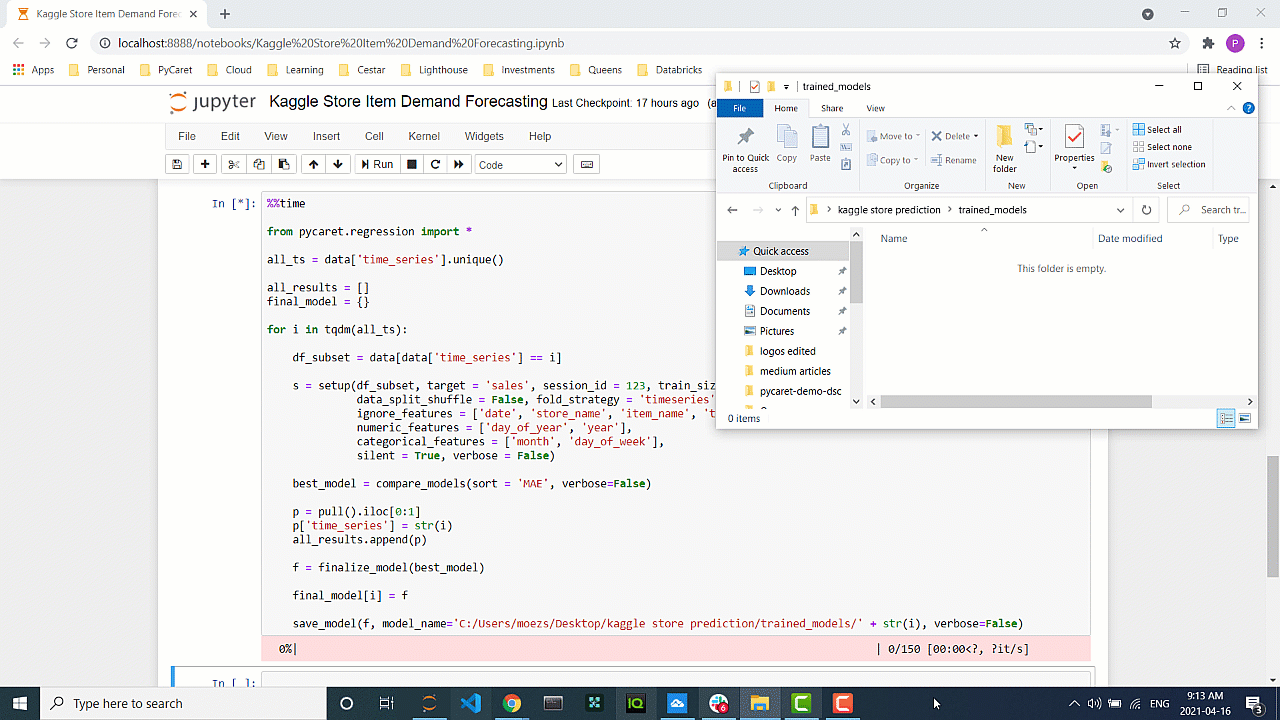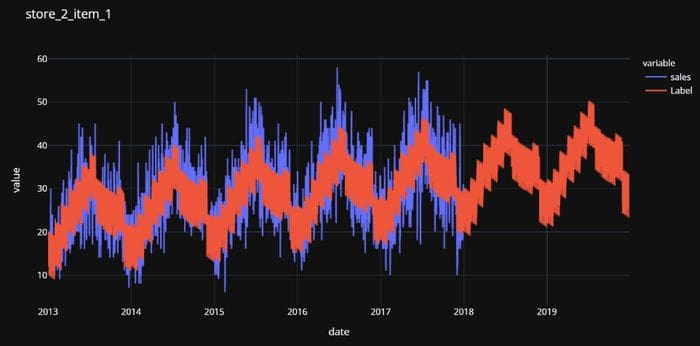# Multiple Time Series Forecasting with PyCaret

A step-by-step tutorial to forecast multiple time series with PyCaret.

By Moez Ali, Founder & Author of PyCaretPyCaret — An open-source, low-code machine learning library in Python

### PyCaret

PyCaret is an open-source, low-code machine learning library and end-to-end model management tool built-in Python for automating machine learning workflows. It is incredibly popular for its ease of use, simplicity, and ability to build and deploy end-to-end ML prototypes quickly and efficiently.

PyCaret is an alternate low-code library that can be used to replace hundreds of lines of code with few lines only. This makes the experiment cycle exponentially fast and efficient.

PyCaret is simple and easy to use. All the operations performed in PyCaret are sequentially stored in a Pipeline that is fully automated for deployment. Whether it’s imputing missing values, one-hot-encoding, transforming categorical data, feature engineering, or even hyperparameter tuning, PyCaret automates all of it.

This tutorial assumes that you have some prior knowledge and experience with PyCaret. If you haven’t used it before, no problem — you can get a quick headstart through these tutorials:

### RECAP

In my last tutorial, I have demonstrated how you can use PyCaret to forecast time-series data using Machine Learning through PyCaret Regression Module. If you haven’t read that yet, you can read Time Series Forecasting with PyCaret Regression Module tutorial before continuing with this one, as this tutorial builds upon some important concepts covered in the last tutorial.

### Installing PyCaret

Installing PyCaret is very easy and takes only a few minutes. We strongly recommend using a virtual environment to avoid potential conflicts with other libraries.

PyCaret’s default installation is a slim version of pycaret which only installs hard dependencies that are listed here.

``````# install slim version (default)
pip install pycaret# install the full version
pip install pycaret[full]``````

When you install the full version of pycaret, all the optional dependencies as listed here are also installed.

### 👉 PyCaret Regression Module

PyCaret Regression Module is a supervised machine learning module used for estimating the relationships between a dependent variable (often called the ‘outcome variable’, or ‘target’) and one or more independent variables (often called ‘features’, or ‘predictors’).

The objective of regression is to predict continuous values such as sales amount, quantity, temperature, number of customers, etc. All modules in PyCaret provide many pre-processing features to prepare the data for modeling through the setup function. It has over 25 ready-to-use algorithms and several plots to analyze the performance of trained models.

### 👉 Dataset

For this tutorial, I will show the end-to-end implementation of multiple time-series data forecasting, including both the training as well as predicting future values.

I have used the Store Item Demand Forecasting Challenge dataset from Kaggle. This dataset has 10 different stores and each store has 50 items, i.e. total of 500 daily level time series data for five years (2013–2017).Sample Dataset

### 👉 Load and prepare the data

``````# read the csv file
import pandas as pd
data['date'] = pd.to_datetime(data['date'])# combine store and item column as time_series
data['store'] = ['store_' + str(i) for i in data['store']]
data['item'] = ['item_' + str(i) for i in data['item']]
data['time_series'] = data[['store', 'item']].apply(lambda x: '_'.join(x), axis=1)
data.drop(['store', 'item'], axis=1, inplace=True)# extract features from date
data['month'] = [i.month for i in data['date']]
data['year'] = [i.year for i in data['date']]
data['day_of_week'] = [i.dayofweek for i in data['date']]
data['day_of_year'] = [i.dayofyear for i in data['date']]data.head()``````Samples rows from data

``````# check the unique time_series
data['time_series'].nunique()
>>> 500``````

### 👉 Visualize time-series

``````# plot multiple time series with moving avgs in a loopimport plotly.express as pxfor i in data['time_series'].unique():
subset = data[data['time_series'] == i]
subset['moving_average'] = subset['sales'].rolling(30).mean()
fig = px.line(subset, x="date", y=["sales","moving_average"], title = i, template = 'plotly_dark')
fig.show()``````store_1_item_1 time series and 30-day moving averagestore_2_item_1 time series and 30-day moving average

### 👉 Start the training process

Now that we have the data ready, let’s start the training loop. Notice that `verbose = False` in all functions to avoid printing results on the console while training.

The code below is a loop around `time_series` column we created during the data preparatory step. There are a total of 150 time series (10 stores x 50 items).

Line 10 below is filtering the dataset for `time_series` variable. The first part inside the loop is initializing the `setup` function, followed by `compare_models` to find the best model. Line 24–26 captures the results and appends the performance metrics of the best model in a list called `all_results`. The last part of the code uses the `finalize_model` function to retrain the best model on the entire dataset including the 5% left in the test set and saves the entire pipeline including the model as a pickle file.

https://gist.github.com/moezali1/f258195ba1c677654abffb0d1acb2cc0

We can now create a data frame from `all_results` list. It will display the best model selected for each time series.

``````concat_results = pd.concat(all_results,axis=0)sample_rows from concat_results

### Training Process 👇Training process

### 👉 Generate predictions using trained models

Now that we have trained models, let’s use them to generate predictions, but first, we need to create the dataset for scoring (X variables).

``````# create a date range from 2013 to 2019
all_dates = pd.date_range(start='2013-01-01', end = '2019-12-31', freq = 'D')# create empty dataframe
score_df = pd.DataFrame()# add columns to dataset
score_df['date'] = all_dates
score_df['month'] = [i.month for i in score_df['date']]
score_df['year'] = [i.year for i in score_df['date']]
score_df['day_of_week'] = [i.dayofweek for i in score_df['date']]
score_df['day_of_year'] = [i.dayofyear for i in score_df['date']]score_df.head()``````sample rows from score_df dataset

Now let’s create a loop to load the trained pipelines and use the `predict_model` function to generate prediction labels.

``````from pycaret.regression import load_model, predict_modelall_score_df = []for i in tqdm(data['time_series'].unique()):
l = load_model('trained_models/' + str(i), verbose=False)
p = predict_model(l, data=score_df)
p['time_series'] = i
all_score_df.append(p)concat_df = pd.concat(all_score_df, axis=0)samples rows from concat_df

We will now join the `data`and `concat_df` .

``````final_df = pd.merge(concat_df, data, how = 'left', left_on=['date', 'time_series'], right_on = ['date', 'time_series'])sample rows from final_df

We can now create a loop to see all plots.

``````for i in final_df['time_series'].unique()[:5]:
sub_df = final_df[final_df['time_series'] == i]

import plotly.express as px
fig = px.line(sub_df, x="date", y=['sales', 'Label'], title=i, template = 'plotly_dark')
fig.show()``````store_1_item_1 actual sales and predicted labelsstore_2_item_1 actual sales and predicted labels

I hope that you will appreciate the ease of use and simplicity in PyCaret. In less than 50 lines of code and one hour of experimentation, I have trained over 10,000 models (25 estimators x 500 time series) and productionalized 500 best models to generate predictions.

### Coming Soon!

Next week I will be writing a tutorial on unsupervised anomaly detection on time-series data using PyCaret Anomaly Detection Module. Please follow me on MediumLinkedIn, and Twitter to get more updates.

There is no limit to what you can achieve using this lightweight workflow automation library in Python. If you find this useful, please do not forget to give us ⭐️ on our GitHub repository.

### Want to learn about a specific module?

Click on the links below to see the documentation and working examples.

Bio: Moez Ali is a Data Scientist, and is Founder & Author of PyCaret.

Original. Reposted with permission.

Related: